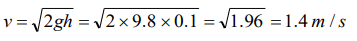## Work, Energy and Power Questions and Answers Part-11

1. If the increase in the kinetic energy of a body is 22%, then the increase in the momentum will be
a) 22%
b) 44%
c) 10%
d) 300%

Explanation: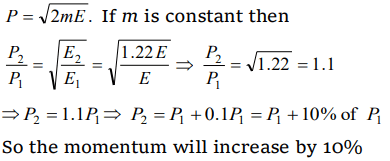2. If a body of mass 200 g falls from a height 200 m and its total P.E. is converted into K.E. at the point of contact of the body with earth surface, then what is the decrease in P.E. of the body at the contact (g = 10 $m\diagup s^{2}$  )
a) 200 J
b) 400 J
c) 600 J
d) 900 J

Explanation: $\triangle$ U = mgh = 0.2 * 10 * 200 = 400 J
Gain in K.E. = decrease in P.E. = 400 J

3. If momentum is increased by 20%, then K.E. increases by
a) 44%
b) 55%
c) 66%
d) 77%

Explanation:4.The kinetic energy of a body of mass 2 kg and momentum of 2 Ns is
a) 1 J
b) 2 J
c) 3 J
d) 4 J

Explanation: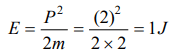5. The decrease in the potential energy of a ball of mass 20 kg which falls from a height of 50 cm is.
a) 968 J
b) 98 J
c) 1980 J
d) None of these

Explanation: $\triangle$ U = mgh = 20 * 9.8 * 0.5 = 98 J

6. An object of 1 kg mass has a momentum of 10 kg m/sec then the kinetic energy of the object will be
a) 100 J
b) 50 J
c) 1000 J
d) 200 J

Explanation: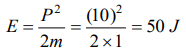7. A ball is released from certain height. It loses 50% of its kinetic energy on striking the ground. It will attain a height again equal to
a) One fourth the initial height
b) Half the initial height
c)Three fourth initial height
d) None of these

Explanation: Because 50% loss in kinetic energy will affect its potential energy and due to this ball will attain only half of the initial height

8. A 0.5 kg ball is thrown up with an initial speed 14 m/s and reaches a maximum height of 8.0m. How much energy is dissipated by air drag acting on the ball during the ascent
a) 19.6Joule
b) 4.9 Joule
c) 10 Joule
d) 9.8 Joule

Explanation:9. An ice cream has a marked value of 700 kcal. How many kilowatt- hour of energy will it deliver to the body as it is digested
a) 0.81kWh
b) 0.90kWh
c) 1.11kWh
d) 0.71kWh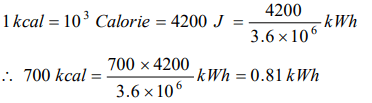10.What is the velocity of the bob of a simple pendulum at its mean position, if it is able to rise to vertical height of 10 cm ( take g=9.8 $m\diagup s^{2}$  )